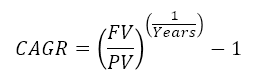The formula for Compound Annual Growth Rate (CAGR) is very useful for investment analysis. It may also be referred to as the annualized rate of return or annual percent yield or effective annual rate, depending on the algebraic form of the equation. Many investments such as stocks have returns that can vary wildly. The CAGR formula allows you to calculate a "smoothed" rate of return that you can use to compare to other investments.

## How to Calculate CAGR

The CAGR formula is commonly defined as CAGR = (End Value/Start Value)^(1/Years)-1.

When you know the overall Growth Rate, (FV-PV)/PV, for an investment over a period of Days, you can calculate the CAGR using the formula CAGR = (1+Growth Rate)^(365/Days)-1, where (End Value / Start Value)=(1+Growth Rate) and (1/Years)=(365/Days).

Likewise, when you know the rate per compound period (r) and the number of compound periods per year (n), you can calculate the effective annual rate using APY = CAGR = (1+r)^n-1.

The CAGR can also be used for the annualized return on investment = CAGR = (1+ROI)^(365/Days)-1 where ROI may be defined as (Revenue-Costs)/Costs.

## Online CAGR Calculator

You can use this online calculator to perform quick calculations, or you can download the spreadsheet to see how to use the CAGR formula in Excel.

### CAGR Calculator, (FV/PV)^(1/Years)-1

 Start or Present Value, PV Ending or Future Value, FV Years CAGR % Leave one field blank to solve for that value.

For: Excel 2007 or later & Excel for iPad/iPhone
License: Personal Use (not for distribution or resale)

## How to Calculate CAGR in Excel

You can calculate CAGR in Excel using the RATE function: CAGR = RATE(Years,,-PV,FV).

The RATE, PV, FV and NPER functions in Excel can be used to calculate each of the four variables associated with the CAGR formula. This is demonstrated in the CAGR_1 tab within the Excel file and the formulas below.

CAGR = RATE(Years,,-PV,FV)

PV = PV(CAGR,Years,,-FV)

FV = FV(CAGR,Years,-PV)

Years = NPER(CAGR,,-PV,FV)

## CAGR Calculation in Excel Using Dates

If you know the total days instead of years, you can substitute 365/Days for 1/Years in the CAGR formula. In Excel, to calculate the Days you only need to subtract the Start Date from the End Date. The following calculator is an embedded Excel spreadsheet. If you have a compatible browser, then you can use this calculator online. To download the Excel file, see the link above.

## The CAGR Formula Explained

The CAGR formula is a way of calculating the Annual Percentage Yield, APY = (1+r)^n-1, where r is the rate per period and n is the number of compound periods per year. For an investment, the period may be shorter or longer than a year, so n is calculated as 1/Years or 365/Days, depending on whether you want to specify the period in Years or Days. The rate per period (r) is the overall growth rate for the investment period.

To understand the CAGR Formula, let's first start by defining Growth as simply End Value minus Start Value.

Growth = End Value - Start Value

Example: If an investment of \$2000 grows to a value of \$5000, the growth is \$5000 - \$2000 = \$3000.

That's pretty simple, and so is the Growth Rate, calculated as the Growth divided by the Start Value:

Growth Rate = Growth / Start Value = End Value / Start Value - 1

Example: Continuing the above example, the Growth Rate is \$3000 / \$2000 = 1.5 or 150%

Note: We could also use Return on Investment (ROI) for the Growth Rate if we wanted to account for interest earned, dividends, fees and commissions, or a series of investments. When using ROI, Growth = Net Profit and Start Value = Total Investment.

The next step is to calculate the annualized compound growth rate by compounding the growth rate over the number of years that we had our investment, using the APY formula (substituting Growth Rate for r and 1/Years for n).

APY = (1 + r ) ^ ( n ) - 1

APY = (1 + Growth Rate ) ^ ( 1 / Years ) - 1

Example: Continuing the above example, if our investment was growing for a period of 3 years, the annualized growth rate = (1+150%)^(1/3)-1 = 35.72%

Next, we just do some simple algebra and substitute End Value / Start Value - 1 for Growth Rate and Eureka! We have the CAGR formula.

APY = CAGR = (End Value / Start Value) ^ ( 1 / Years ) - 1

Example: We can see that the APY calculated in the previous step is the same as the CAGR = (5000/2000)^(1/3)-1 = 35.72%

### References

Disclaimer: This information on this page is for educational purposes only. We do not guarantee the results or the applicability to your unique financial situation. You should seek the advice of qualified professionals regarding financial decisions.